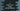# C program to swap two numbers using call by reference### Write a program to swap two numbers using call by reference :

In this example, we will learn how to swap two numbers using call by reference or by using pointers. Let’s take a look into the program :

### C program :

``````#include <stdio.h>

//4
{
int temp;

}

int main()
{
//1
int firstNumber, secondNumber;

//2
printf("Enter two numbers you want to swap : ");
scanf("%d%d", &firstNumber, &secondNumber);

//3
swapNumbers(&firstNumber, &secondNumber);

//5
printf("First and Second numbers after swapped = %d , %d ", firstNumber, secondNumber);
}
``````

### Explanation :

_The commented numbers in the above program denotes the steps below : _

1. Create two variables firstNumber and secondNumber to store the first and second values.
2. Ask the user to enter both numbers and save the values in firstNumber and secondNumber.
3. Swap numbers by passing the address of firstNumber and secondNumber.
4. void swapNumbers(int *firstNumberAddress, int *secondNumberAddress) function is used to swap both numbers . It takes address of both numbers and swap the contents of these addresses using temp variable.
5. After the swap is completed, print the values of both numbers.